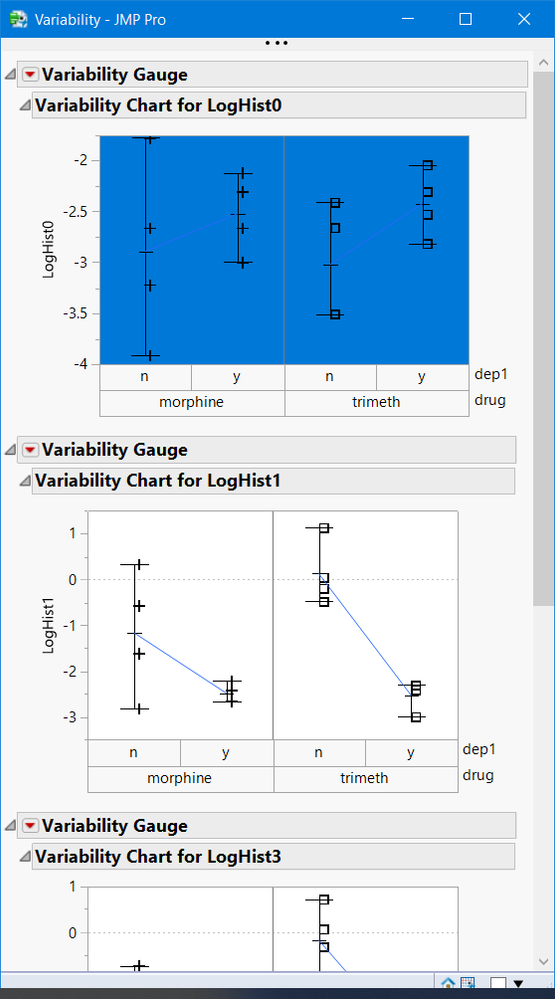Choose Language Hide Translation Bar
Highlighted

## Variability Plot Grouping

Hi,

I have a bunch of variability plots to show on a single window with same columns grouping. How will I just define the x_grouping at the very top, so that I can change the grouping in only one place. For example, I want to define grouping by :xA, :xB, and ;xC outside the function "variability chart ( )", so that I can change grouping columns in one place only. Thanks.

``````Names Default To Here( 1 );

dt = Current Data Table( );

/*

A general grouping variable for X grouping.

*/

New Window(
"Variability",
V List Box(
Variability Chart(
Y( :Y1 ),
X( :xA, :xB, :xC ),
),
Variability Chart(
Y( :Y1 ),
X( :xA, :xB, :xC ),
Connect Cell Means( 1 )
)
)
);``````

1 ACCEPTED SOLUTION

Accepted Solutions
Highlighted

## Re: Variability Plot Grouping

@RA

You can create multiple variability plots with one Variability Plot() platform statement

``````Names Default To Here( 1 );
dt = Open( "\$SAMPLE_DATA/Dogs.jmp" );

New Window(
"Variability",
V List Box(
vc = dt << Variability Chart(
Y( :LogHist0, :LogHist1, :LogHist3, :LogHist5 ),
X( :drug, :dep1 ),
Connect Cell Means( 1 ),
Std Dev Chart( 0 )
)
) //end VListBox
);

show(vc);
//vc = {Variability Chart[], Variability Chart[], Variability Chart[], Variability Chart[]};

//you can reference and change the the plots, see below

report(vc)[FrameBox(1)] << select;

``````4 REPLIES 4
Highlighted

## Re: Variability Plot Grouping

@RA

You can create multiple variability plots with one Variability Plot() platform statement

``````Names Default To Here( 1 );
dt = Open( "\$SAMPLE_DATA/Dogs.jmp" );

New Window(
"Variability",
V List Box(
vc = dt << Variability Chart(
Y( :LogHist0, :LogHist1, :LogHist3, :LogHist5 ),
X( :drug, :dep1 ),
Connect Cell Means( 1 ),
Std Dev Chart( 0 )
)
) //end VListBox
);

show(vc);
//vc = {Variability Chart[], Variability Chart[], Variability Chart[], Variability Chart[]};

//you can reference and change the the plots, see below

report(vc)[FrameBox(1)] << select;

``````Highlighted

## Re: Variability Plot Grouping

@gzmorgan0
Can you explain the purpose of these two statements a bit more?

``````Show( vc );
//vc = {Variability Chart[], Variability Chart[], Variability Chart[], Variability Chart[]};
Report( vc )[FrameBox( 1 )] << select;``````
Highlighted

## Re: Variability Plot Grouping

@RA ,

When scripting, it is useful to create variable references to the objects, in your case the 4 variability charts.  I do not know your JSL experience, so those statements are showing you how to create a variable reference to the varaibility charts.

• vc = dt << Variability Chart()  sets up a reference to a list of the varaibiity charts created by  this statement
• you can referencs them in a for loop   For( i=1, i<=nitems(vc), i++, vc[i] <<   ...   or report( vc[i]  ) << ...
• some options you can change all  vc << Std Dev(1)
``````//change var chart
report(vc)[FrameBox(1)] << {Background Color( 32)} ;

//change all var charts
vc << Connect Cell Means(0);
wait(2);
vc << Connect Cell Means(1);
``````

My goal was inform you of this capability just in case you were not aware, that is, I was anticipating a next question.  Sorry if it was unclear.

Highlighted

## Re: Variability Plot Grouping

I am new to jsl. This referencing tip is highly appreciated.
Article Labels